## Abstract

We present a Lagrangian formulation for coupled thermal analysis of quasi and fully incompressible flows and fluid-structure interaction (FSI) problems that has excellent mass preservation features. The success of the formulation lays on a residual-based stabilized expression of the mass balance equation obtained using the Finite Calculus (FIC) method. The governing equations are discretized with the FEM using simplicial elements with equal linear interpolation for the velocities, the pressure and the temperature. The merits of the formulation in terms of reduced mass loss and overall accuracy are verified in the solution of 2D and 3D adiabatic and thermally-coupled quasi-incompressible free-surface flow problems using the Particle Finite Element Method (PFEM). Examples include the sloshing of water in a tank and the falling of a water sphere and a cylinder into a tank containing water.

Keywords: Particle Finite Element Method, Coupled Thermal Analysis, Quasi and Fully Incompressible

## 1 INTRODUCTION

The analysis of thermally coupled flows and their interaction with structures is relevant in many fields of engineering. In this work we present a Lagrangian numerical technique for solving this kind of problems for quasi and fully incompressible fluids using the Particle Finite Element Method (PFEM, www.cimne.com/pfem).

The PFEM treats the mesh nodes in the analysis domain as particles which can freely move and even separate from the domain representing, for instance, the effect of water drops or cutting particles in drilling problems. A mesh connects the nodes discretizing the domain where the governing equations are solved using a stabilized FEM. Examples of application of PFEM to problems in fluid and solid mechanics including fluid-structure interaction (FSI) situations can be found in [4,5,6,8,9,10,11,12,13,14,15,16,17,18,19,28,29,35,36,39,40,41,42,43]. Early attempts of the PFEM for solving thermally coupled flows were reported in [1,2].

In Lagrangian analysis procedures (such as PFEM) the motion of the fluid particles is tracked during the transient solution. Hence, the convective terms vanish in the momentum and heat transfer equations and no numerical stabilization is needed for treating those terms. Two other sources of mass loss, however, remain in the numerical solution of Lagrangian flows, i.e. that due to the treatment of the incompressibility constraint by a stabilized numerical method, and that induced by the inaccuracies in tracking the flow particles and, in particular, the free surface.

In this work the PFEM equations for analysis of thermally coupled flows and FSI problems are derived using the stabilized formulation based in the Finite Calculus (FIC) method proposed by Oñate et al. [20,21,22,23,24,25,26,27,30,31,32,37,38,39] that has excellent mass preservation features.

The lay-out of the paper is the following. In the next section we present the basic equations for conservation of linear momentum, mass and heat transfer for a quasi-incompressible fluid in a Lagrangian framework. A full incompressible fluid can be considered as a particular limit case of the former. Next we derive the stabilized FIC form of the mass balance equation. Then the finite element discretization using simplicial element with equal order approximation for the velocity, the pressure and the temperature is presented and the relevant matrices and vectors of the discretized problem are given. Details of the implicit solution of the Lagrangian FEM equations in time using a Newton iterative scheme are presented. The relevance of the bulk stiffness terms in the tangent matrix for enhancing the convergence and accuracy of the iterative solution scheme is discussed. The basic steps of the PFEM for solving coupled free-surface FSI problems are described.

The efficiency and accuracy of the PFEM technique are verified by solving a set of adiabatic and thermally coupled quasi-incompressible free surface flow problems in two (2D) and three (3D) dimensions with the PFEM. The adiabatic problems are the sloshing of water in a tank and the penetration of a water sphere into a cylindrical tank containing water. The thermally coupled problems considered are the extended 2D version of the adiabatic cases. The excellent performance of the numerical method proposed in terms of mass conservation and general accuracy is highlighted.

## 2 GOVERNING EQUATIONS

We write the governing equations for a quasi-incompressible Newtonian flow problem in the Lagrangian description as follows [3,46].

### Momentum equations

 $\rho {\frac {Dv_{i}}{Dt}}-{\partial \sigma _{ij} \over \partial x_{j}}-b_{i}=0\quad ,\quad i,j=1,n_{s}\quad {\hbox{in }}\Omega$
(1)

In Eq.(1), ${\textstyle \Omega }$ is the analysis domain with boundary ${\textstyle \Gamma }$, ${\textstyle v_{i}}$ and ${\textstyle b_{i}}$ are the velocity and body force components along the ${\textstyle i}$th Cartesian axis, ${\textstyle \rho }$ is the density, ${\textstyle n_{s}}$ is the number of space dimensions (i.e. ${\textstyle n_{s}=3}$ for 3D problems) and ${\textstyle \sigma _{ij}}$ are the Cauchy stresses that are split in the deviatoric (${\textstyle s_{ij}}$) and pressure (${\textstyle p}$) components as

 $\sigma _{ij}=s_{ij}+p\delta _{ij}$
(2)

where ${\textstyle \delta _{ij}}$ is the Kronecker delta. Note that the pressure is assumed to be positive for a tension state. Summation of terms with repeated indices is assumed in Eq.(1) and in the following, unless otherwise specified.

The relationship between the deviatoric stresses ${\textstyle s_{ij}}$ and the strain rates ${\textstyle \varepsilon _{ij}}$ has the standard form for a Newtonian fluid,

 $s_{ij}=2\mu \left(\varepsilon _{ij}-{1 \over 3}\varepsilon _{v}\delta _{ij}\right)\quad {\hbox{with}}\quad \varepsilon _{ij}={1 \over 2}\left({\partial v_{i} \over \partial x_{j}}+{\partial v_{j} \over \partial x_{i}}\right)$
(3)

where ${\textstyle \mu }$ is the viscosity and ${\textstyle \varepsilon _{v}}$ is the volumetric strain rate defined as ${\textstyle \varepsilon _{v}=\varepsilon _{ii}={\partial v_{i} \over \partial x_{i}}}$.

### Mass balance equation

The standard mass balance equation for a quasi-incompressible fluid can be written as [3,7,46]

 $r_{v}=0$
(4a)

with

 $r_{v}:=-{\frac {1}{c^{2}}}{\frac {Dp}{Dt}}+\rho \varepsilon _{v}$
(4b)

In Eq.(4b) ${\textstyle c}$ is the speed of sound in the fluid. For a fully incompressible fluid ${\textstyle c=\infty }$ and Eq.(4a) simplifies to the standard form, ${\textstyle \varepsilon _{v}=0}$. In our work we will retain the quasi-incompressible form of ${\textstyle r_{v}}$ of Eq.(4b) for convenience.

### Thermal balance

 $\rho c{\frac {DT}{Dt}}-{\partial \over \partial x_{i}}\left(k{\partial T \over \partial x_{i}}\right)+Q=0\quad i=1,n_{s}\quad {\hbox{in }}\Omega$
(5)

where ${\textstyle T}$ is the temperature, ${\textstyle c}$ is the thermal capacity, ${\textstyle k}$ is the heat conductivity and ${\textstyle Q}$ is the heat source.

### Mechanical problem

The boundary conditions at the Dirichlet (${\textstyle \Gamma _{v}}$) and Neumann (${\textstyle \Gamma _{t}}$) boundaries with ${\textstyle \Gamma =\Gamma _{v}\cup \Gamma _{t}}$ are

 $v_{i}-v_{i}^{p}=0\qquad {\hbox{on }}\Gamma _{v}$
(6)

 $\sigma _{ij}n_{j}-t_{i}^{p}=0\quad {\hbox{on }}\Gamma _{t}\quad i,j=1,n_{s}$
(7)

where ${\textstyle v_{i}^{p}}$ and ${\textstyle t_{i}^{p}}$ are the prescribed velocities and prescribed tractions on ${\textstyle \Gamma _{v}}$ and ${\textstyle \Gamma _{t}}$, respectively and ${\textstyle n_{j}}$ are the components of the unit normal vector to the boundary [3,7,46].

### Thermal problem

 $\phi -\phi ^{p}=0\quad {\hbox{on }}\Gamma _{\phi }$ (8) $k{\partial \phi \over \partial n}+q_{n}^{p}=0\quad {\hbox{on }}\Gamma _{q}$ (9)

where ${\textstyle \phi ^{p}}$ and ${\textstyle q_{n}^{p}}$ are the prescribed temperature and the prescribed normal heat flux at the boundaries ${\textstyle \Gamma _{\phi }}$ and ${\textstyle \Gamma _{q}}$, respectively and ${\textstyle n}$ is the direction normal to the boundary.

Remark 1. The term ${\textstyle {\frac {Dv_{i}}{Dt}}}$ in Eq.(1) is the material derivative of the ${\textstyle i}$th velocity component ${\textstyle v_{i}}$. This term is typically computed in a Lagrangian framework as

 ${\frac {Dv_{i}}{Dt}}={\frac {{}^{n+1}v_{i}-{}^{n}v_{i}}{\Delta t}}$
(10)

with

 ${}^{n+1}v_{i}:=v_{i}({}^{n+1}{\bf {x}},{}^{n+1}t)\quad ,\quad {}^{n}v_{i}:=v_{i}({}^{n}{\bf {x}},{}^{n}t)$
(11)

where ${\textstyle {}^{n}v_{i}}$ is the velocity of the material point that has the position ${\textstyle {}^{n}{\bf {x}}}$ at time ${\textstyle t={}^{n}t}$, where ${\textstyle {\bf {x}}}$ is the coordinates vector in a fixed Cartesian system [3,7,46].

## 3 STABILIZED MASS BALANCE EQUATION

In this work we will use the second order FIC form of the mass balance equation in space for a quasi-incompressible fluid [37,38], as well as the first order FIC form of the mass balance equation in time. These forms have the following expressions:

### Second order FIC mass balance equation in space

 $r_{v}+{\frac {h_{i}^{2}}{12}}{\frac {\partial ^{2}r_{v}}{\partial x_{i}^{2}}}=0\qquad {\hbox{in }}\Omega \qquad i=1,n_{s}$
(12a)

### First order FIC mass balance equation in time

 $r_{v}+{\frac {\delta }{2}}{\frac {Dr_{v}}{Dt}}=0\qquad {\hbox{in }}\Omega$
(12b)

Eq.(12a) is obtained by expressing the balance of mass in a rectangular domain of finite size with dimensions ${\textstyle h_{1}\times h_{2}}$ (for 2D problems), where ${\textstyle h_{i}}$ are arbitrary distances, and retaining up to third order terms in the Taylor series expansions used for expressing the change of mass within the balance domain.

Eq.(12b), on the other hand, is obtained by expressing the balance of mass in a space-time domain of infinitesimal length in space and finite dimension ${\textstyle \delta }$ in time . The derivation of Eqs.(12) for a 1D problem are shown in .

The FIC terms in Eqs.(12) play the role of space and time stabilization terms respectively. In the discretized problem, the space dimensions ${\textstyle h_{i}}$ and the time dimension ${\textstyle \delta }$ are related to characteristic element dimensions and the time step increment, respectively as it will be explained later. Note that for ${\textstyle h_{i}=0}$ and ${\textstyle \delta =0}$ the standard infinitesimal form of the mass balance equation, ${\textstyle r_{v}=0}$, is obtained.

After some transformations the stabilized mass balance equation (12a) is written as 

 $-{\frac {1}{\kappa }}{\frac {Dp}{Dt}}+\varepsilon _{v}-{\frac {\tau }{c^{2}}}{\frac {D^{2}p}{Dt^{2}}}+\tau {\partial {\hat {r}}_{m_{i}} \over \partial x_{i}}=0$
(13)

where ${\textstyle \tau }$ is a stabilization parameter given by 

 $\tau =\left({\frac {8\mu }{h^{2}}}+{\frac {2\rho }{\delta }}\right)^{-1}$
(14)

and ${\textstyle {\hat {r}}_{m_{i}}}$ is a static momentum term defined as

 ${\hat {r}}_{m_{i}}={\partial \over \partial x_{j}}(2\mu \varepsilon _{ij})+{\frac {\partial p}{\partial x_{i}}}+b_{i}$
(15)

Eq.(13) is used as the starting point for deriving the stabilized FEM formulation as explained in the following sections.

## 4 VARIATIONAL EQUATIONS

### 4.1 Momentum equations

Multiplying Eq.(1) by arbitrary test functions ${\textstyle w_{i}}$ with dimensions of velocity and integrating over the analysis domain ${\textstyle \Omega }$ gives the weighted residual form of the momentum equations as [3,7,46]

 $\int _{\Omega }w_{i}\left(\rho {\frac {Dv_{i}}{Dt}}-{\partial \sigma _{ij} \over \partial x_{j}}-b_{i}\right)d\Omega =0$
(16)

Integrating by parts the term involving ${\textstyle \sigma _{ij}}$ and using the Neumann boundary conditions (7) yields the weak variational form of the momentum equations as

 $\int _{\Omega }w_{i}\rho {\frac {Dv_{i}}{Dt}}d\Omega +\int _{\Omega }\delta \varepsilon _{ij}\sigma _{ij}d\Omega -\int _{\Omega }w_{i}b_{i}d\Omega -\int _{\Gamma _{t}}w_{i}t_{i}^{p}d\Gamma =0$
(17)

where ${\textstyle \delta \varepsilon _{ij}={\partial w_{i} \over \partial x_{j}}+{\partial w_{j} \over \partial x_{i}}}$ is an arbitrary (virtual) strain rate field. Eq.(17) is the standard form of the principle of virtual power [3,7,46].

Substituting the expression of the stresses from Eq.(2) into (17) gives

 $\int _{\Omega }\!w_{i}\rho {\frac {Dv_{i}}{Dt}}d\Omega +\!\int _{\Omega }\left[\delta \varepsilon _{ij}2\mu \left(\!\varepsilon _{ij}-{\frac {1}{3}}\varepsilon _{v}\delta _{ij}\!\right)+\delta \varepsilon _{v}p\right]d\Omega -\!\int _{\Omega }w_{i}b_{i}d\Omega -\!\int _{\Gamma _{t}}w_{i}t_{i}^{p}d\Gamma =0$
(18)

Eq.(18) can be written in matrix form as

 $\displaystyle \int _{\Omega }{\bf {w}}^{T}\rho {\frac {D{\bf {v}}}{Dt}}d\Omega +\!\int _{\Omega }\delta {\boldsymbol {\varepsilon }}^{T}{\bf {D}}{\boldsymbol {\varepsilon }}d\Omega +\!\int _{\Omega }\delta {\boldsymbol {\varepsilon }}^{T}{\bf {m}}pd\Omega -\int _{\Omega }{\bf {w}}^{T}{\bf {b}}d\Omega -\!\int _{\Gamma _{t}}{\bf {w}}^{T}{\bf {t}}^{p}d\Gamma =0$
(19)

In Eq.(19) ${\textstyle {\bf {w}},{\bf {v}},{\boldsymbol {\varepsilon }}}$ and ${\textstyle \delta {\boldsymbol {\varepsilon }}}$ are vectors containing the test functions, the velocities, the strain rates and the virtual strain rates respectively; ${\textstyle {\bf {b}}}$ and ${\textstyle {\bf {t}}^{p}}$ are body force and surface traction vectors, respectively; ${\textstyle {\bf {D}}}$ is the viscous constitutive matrix and ${\textstyle {\bf {m}}}$ is an auxiliary vector. These vectors are defined as (for 3D problems)

 ${\begin{array}{l}{\textbf {w}}=[w_{1},w_{2},w_{3}]^{T}\quad ,\quad {\textbf {v}}=[v_{1},v_{2},v_{3}]^{T}\quad ,\quad {\textbf {b}}=[b_{1},b_{2},b_{3}]^{T}\quad ,\quad {\textbf {t}}^{p}=[t_{1}^{p},t_{2}^{p},t_{3}^{p}]^{T}\\[.5cm]{\boldsymbol {\varepsilon }}=[\varepsilon _{11},\varepsilon _{22},\varepsilon _{33},\varepsilon _{12},\varepsilon _{13},\varepsilon _{23}]^{T}\quad ,\quad \delta {\boldsymbol {\varepsilon }}=[\delta \varepsilon _{11},\delta \varepsilon _{22},\delta \varepsilon _{33},\delta \varepsilon _{12},\delta \varepsilon _{13},\delta \varepsilon _{23}]^{T}\\[.5cm]{\bf {D}}=\mu \left[{\begin{array}{cccccc}4/3&-2/3&-2/3&0&0&0\\&4/3&-2/3&0&0&0\\&&4/3&0&0&0\\&&&2&0&0\\{Sym.}&&&&2&0\\&&&&&2\\\end{array}}\right]\qquad ,\qquad {\bf {m}}=[1,1,1,0,0,0]^{T}\end{array}}$
(20)

### 4.2 Mass balance equations

We multiply Eq.(13) by arbitrary (continuous) test functions ${\textstyle q}$ (with dimensions of pressure) defined over the analysis domain ${\textstyle \Omega }$. Integrating over ${\textstyle \Omega }$ gives

 $\int _{\Omega }-{\frac {q}{\kappa }}{\frac {Dp}{Dt}}d\Omega -\int _{\Omega }q{\frac {\tau }{c^{2}}}{\frac {D^{2}p}{Dt^{2}}}d\Omega +\int _{\Omega }q\varepsilon _{v}d\Omega +\int _{\Omega }q\tau {\partial {\hat {r}}_{m_{i}} \over \partial x_{i}}d\Omega =0$
(21)

Integrating by parts the last integral in Eq.(21) and using (15) gives after some transformations [39,40]

 ${\begin{array}{r}\displaystyle \int _{\Omega }{\frac {q}{\kappa }}{\frac {Dp}{Dt}}d\Omega +\int _{\Omega }q{\frac {\tau }{c^{2}}}{\frac {D^{2}p}{Dt^{2}}}d\Omega -\int _{\Omega }q\varepsilon _{v}d\Omega +\int _{\Omega }\tau {\partial q \over \partial x_{i}}\left({\partial \over \partial x_{i}}(2\mu \varepsilon _{ij})+{\partial p \over \partial x_{i}}+b_{i}\right)d\Omega \\\displaystyle -\int _{\Gamma _{t}}q\tau \left[\rho {\frac {Dv_{n}}{Dt}}-{\frac {2}{h_{n}}}\left(2\mu {\partial v_{n} \over \partial n}+p-t_{n}\right)\right]d\Gamma =0\end{array}}$
(22)

Expression (24) holds for 2D and 3D problems. The terms involving the first and second material time derivative of the pressure and the boundary term in Eq.(24) are important for preserving the conservation of mass in free-surface flow problems [10,41].

### 4.3 Thermal balance equation

Application of the standard weighted residual method to the heat balance equations (5) and (9) leads, after standard operations, to [7,44]

 $\int _{\Omega }{\hat {w}}\rho c{\partial T \over \partial t}d\Omega +\int _{\Omega }{\partial {\hat {w}} \over \partial x_{i}}k{\partial T \over \partial x_{i}}d\Omega -\int _{\Omega }{\hat {w}}Qd\Omega +\int _{\Gamma _{q}}{\hat {w}}q_{n}^{p}d\Gamma =0$
(23)

where ${\textstyle {\hat {w}}}$ are the space weighting functions for the temperature.

## 5 FEM DISCRETIZATION

We discretize the analysis domain into finite elements with ${\textstyle n}$ nodes in the standard manner leading to a mesh with a total number of ${\textstyle N_{e}}$ elements and ${\textstyle N}$ nodes. In our work we will choose simple 3-noded linear triangles (${\textstyle n=3}$) for 2D problems and 4-noded tetrahedra (${\textstyle n=4}$) for 3D problems with local linear shape functions ${\textstyle N_{i}^{e}}$ defined for each node ${\textstyle i}$ (${\textstyle i=1,n}$) of element ${\textstyle e}$ [34,44]. The velocity components, the pressure and the temperature are interpolated over the mesh in terms of their nodal values in the same manner using the global linear shape functions ${\textstyle N_{j}}$ spanning over the elements sharing node ${\textstyle j}$ (${\textstyle j=1,N}$) [34,44,46]. In matrix form

 ${\bf {v}}={\bf {N}}_{v}{\bar {\bf {v}}}~,~p={\bf {N}}_{p}{\bar {\bf {p}}}\quad ,\quad T={\bf {N}}_{T}{\bar {\bf {T}}}$
(24)

where

 ${\begin{array}{c}\displaystyle {\bar {\bf {v}}}=\left\{{\begin{matrix}{\bar {\bf {v}}}^{1}\\{\bar {\bf {v}}}^{2}\\\vdots \\{\bar {\bf {v}}}^{N}\end{matrix}}\right\}\quad {\hbox{with}}~{\bar {\bf {v}}}^{i}=\left\{{\begin{matrix}{\bar {v}}_{1}^{i}\\{\bar {v}}_{2}^{i}\\{\bar {v}}_{3}^{i}\end{matrix}}\right\}~,~{\bar {\bf {p}}}=\left\{{\begin{matrix}{\bar {p}}^{1}\\{\bar {p}}^{2}\\\vdots \\{\bar {p}}^{N}\end{matrix}}\right\}~,~{\bf {T}}=\left\{{\begin{matrix}{\bar {T}}_{1}\\{\bar {T}}_{2}\\\vdots \\{\bar {T}}^{N}\end{matrix}}\right\}\\\displaystyle {\bf {N}}_{v}=[{\bf {N}}_{1},{\bf {N}}_{2},\cdots ,{\bf {N}}_{N}]^{T}~,~{\bf {N}}_{p}={\bf {N}}_{T}=[{N}_{1},{N}_{2},\cdots ,{N}_{N}]\end{array}}$
(25)

with ${\textstyle {\bf {N}}_{j}=N_{j}{\bf {I}}_{n}}$ where ${\textstyle {\bf {I}}_{n}}$ is the ${\textstyle n\times n}$ unit matrix.

In Eq.(25) vectors ${\textstyle {\bar {v}}}$, ${\textstyle {\bar {p}}}$ and ${\textstyle {\bar {T}}}$ contain the nodal velocities, the nodal pressures and the nodal temperatures for the whole mesh, respectively and the upperindex denotes the nodal value for each vector or scalar magnitude.

Substituting Eqs.(24) into Eqs.(17), (22) and (25) and choosing a Galerkin formulation with ${\textstyle w_{i}=q={\hat {w}}_{i}=N_{i}}$ leads to the following system of algebraic equations

 ${\bf {M}}_{0}{\dot {\bar {\bf {v}}}}+{\bf {K}}{\bar {\bf {v}}}+{\bf {Q}}{\bar {\bf {p}}}-{\bf {f}}_{v}={\bf {0}}$
(26a)

 ${\textbf {M}}_{1}{\dot {\bar {\bf {p}}}}+{\textbf {M}}_{2}{\ddot {\bar {\bf {p}}}}-{\bf {Q}}^{T}{\bar {\bf {v}}}+({\bf {L}}+{\bf {M}}_{b}){\bar {\bf {p}}}-{\bf {f}}_{p}={\bf {0}}$
(26b)

 ${\bf {C}}{\dot {\bar {\bf {T}}}}+{\hat {\bf {L}}}{\bar {\bf {T}}}-{\bf {f}}_{T}={\bf {0}}$
(26c)

where ${\textstyle {\dot {\bar {\bf {a}}}}}$ and ${\textstyle {\ddot {\bar {\bf {a}}}}}$ denote the first and second material time derivatives of the components of a vector ${\textstyle {a}}$. The different matrices and vectors in Eqs.(26) are assembled from the element contributions given in Box 1.

Remark 2. The boundary terms of vector ${\textstyle {\bf {f}}_{p}}$ can be incorporated in the matrices of Eq.(26b). This leads to a non symmetrical set of equations. These boundary terms are computed here iteratively within the incremental solution scheme.

Remark 3. The presence of matrix ${\textstyle {\bf {M}}_{b}}$ in Eq.(26b) allows us to compute the pressure without the need of prescribing its value at the free surface. This eliminates the error introduced when the pressure is prescribed to zero in free boundaries, which leads to considerable mass losses in viscous flows .

Remark 4. For transient problems the stabilization parameter ${\textstyle \tau }$ of Eq.(14) is computed for each element ${\textstyle e}$ using ${\textstyle h=l^{e}}$ and ${\textstyle \delta =\Delta t}$ as

 $\tau =\left({\frac {8\mu }{(l^{e})^{2}}}+{\frac {2\rho }{\Delta t}}\right)^{-1}$
(27)

where ${\textstyle \Delta t}$ is the time step used for the transient solution and ${\textstyle l^{e}}$ is a characteristic element length computed as ${\textstyle l^{e}=2(\Omega ^{e})^{1/n_{s}}}$ where ${\textstyle \Omega ^{e}}$ is the element area (for 3-noded triangles) or volume (for 4-noded tetrahedra). For fluids with heterogeneous material the values of ${\textstyle \mu }$ and ${\textstyle \rho }$ are computed at the element center.

For steady state problems the stabilization parameter is computed with Eq.(27) substituting ${\textstyle \Delta t}$ by ${\textstyle {\frac {l^{e}}{\vert {\bf {v}}^{e}\vert }}}$. The characteristic boundary length ${\textstyle h_{n}}$ in the expression of ${\textstyle {\bf {f}}_{p}}$ (Box 1) has been taken equal to ${\textstyle l^{e}}$ in our computations.

 ${\begin{array}{l}\displaystyle \mathbf {M} _{0_{ij}}^{e}=\int _{\Omega ^{e}}\rho {N}_{i}^{e}{N}_{j}{\bf {I}}_{3}d\Omega ~,~\mathbf {K} _{ij}^{e}=\int _{\Omega ^{e}}\mathbf {B} _{i}^{eT}\mathbf {D} \mathbf {B} _{j}^{e}d\Omega ~,~\mathbf {Q} _{ij}^{e}=\int _{\Omega ^{e}}\mathbf {B} _{i}^{eT}\mathbf {m} {N}_{j}^{e}d\Omega \\[.4cm]\displaystyle {M}_{1_{ij}}^{e}=\int _{\Omega ^{e}}{\frac {1}{\kappa }}{N}_{i}^{e}{N}_{j}^{e}d\Omega ~,~{M}_{2_{ij}}^{e}=\int _{\Omega ^{e}}{\frac {\tau }{c^{2}}}{N}_{i}^{e}{N}_{j}^{e}d\Omega ~,~{M}_{b_{ij}}^{e}=\int _{\Gamma _{t}}{\frac {2\tau }{h_{n}}}{N}_{i}^{e}{N}_{j}^{e}d\Gamma \\[.4cm]\displaystyle {L}_{ij}^{e}=\int _{\Omega ^{e}}\tau ({\boldsymbol {\nabla }}^{T}{N}_{i}^{e}){\boldsymbol {\nabla }}N_{j}^{e}d\Omega ~,~\mathbf {f} _{v_{i}}^{e}=\int _{\Omega ^{e}}\mathbf {N} _{i}^{e}\mathbf {b} d\Omega +\int _{\Gamma _{t}}\mathbf {N} _{i}^{e}{\bf {t}}^{p}d\Gamma \\[.4cm]\displaystyle {f}_{p_{i}}^{e}=\int _{\Gamma _{t}}\tau N_{i}^{e}\left[\rho {\frac {Dv_{n}}{Dt}}-{\frac {2}{h_{n}}}(2\mu \varepsilon _{n}-t_{n})\right]d\Gamma -\int _{\Omega ^{e}}\tau {\boldsymbol {\nabla }}^{T}{N}_{i}^{e}{b}d\Omega \\[.4cm]\displaystyle {C}_{ij}^{e}=\int _{\Omega ^{e}}\rho cN_{i}^{e}N_{j}^{e}d\Omega ~,~{\hat {L}}_{ij}^{e}=\int _{\Omega ^{e}}k({\boldsymbol {\nabla }}^{T}{N}_{i}^{e}){\boldsymbol {\nabla }}N_{j}^{e}d\Omega ~,~\displaystyle {f}_{T_{i}}^{e}=\int _{\Omega ^{e}}N_{i}^{e}Qd\Omega -\int _{\Gamma _{q}}N_{i}^{e}q_{n}^{p}d\Gamma \\[.4cm]{\hbox{with }}i,j=1,n.\\{\hbox{For 3D problems}}\\[.4cm]\displaystyle {\bf {B}}_{i}^{e}=\left[{\begin{matrix}\displaystyle {\partial N_{i}^{e} \over \partial x_{1}}&0&0\\\displaystyle {0}&\displaystyle {\partial N_{i}^{e} \over \partial x_{2}}&0\\\displaystyle {0}&0&\displaystyle {\partial N_{i}^{e} \over \partial x_{3}}\\\displaystyle {\partial N_{i}^{e} \over \partial x_{2}}&\displaystyle {\partial N_{i}^{e} \over \partial x_{1}}&0\\[.25cm]\displaystyle {\partial N_{i}^{e} \over \partial x_{3}}&0&\displaystyle {\partial N_{i}^{e} \over \partial x_{1}}\\[.25cm]\displaystyle {0}&\displaystyle {\partial N_{i}^{e} \over \partial x_{3}}&\displaystyle {\partial N_{i}^{e} \over \partial x_{2}}\end{matrix}}\right]~,~\mathbf {\bf {N}} _{i}^{e}=N_{i}^{e}{\bf {I}}_{3}\quad {\hbox{and}}\quad {\boldsymbol {\nabla }}=\left\{{\begin{matrix}\displaystyle {\partial \over \partial x_{1}}\\\displaystyle {\partial \over \partial x_{2}}\\\displaystyle {\partial \over \partial x_{3}}\end{matrix}}\right\}\end{array}}$ $N_{i}^{e}$: Local shape function of node ${\textstyle i}$ of element ${\textstyle e}$ [34,44]
Box 1. Element form of the matrices and vectors in Eqs.(26)

## 6 TRANSIENT SOLUTION OF THE DISCRETIZED EQUATIONS

Eqs.(26) are solved in time with an implicit Newton-Raphson type iterative scheme [3,7,44,46]. The basic steps within a time increment ${\textstyle [n,n+1]}$ are:

• Initialize variables: ${\textstyle ({}^{n+1}{\bf {x}}^{1},{}^{n+1}{\bar {\bf {v}}}^{1},{}^{n+1}{\bar {\bf {p}}}^{1},{}^{n+1}{\bar {\bf {T}}}^{1},{}^{n+1}{\bar {\bf {r}}}_{m}^{1})\equiv \left\{{}^{n}{\bf {x}},{}^{n}{\bar {\bf {v}}},{}^{n}{\bar {\bf {p}}},{}^{n}{\bar {\bf {T}}},{}^{n}{\bar {\bf {r}}}_{m}\right\}}$.
• Iteration loop: ${\textstyle i=1,NITER}$.

For each iteration.

### Step 1. Compute the nodal velocity increments $\Delta {\bar {v}}$Step 1. Compute the nodal velocity increments Δ v ¯ {\displaystyle \Delta {\bar {v}}}

From Eq.(26a), we deduce

 ${}^{n+1}{\bf {H}}_{v}^{i}\Delta {\bar {\bf {v}}}=-{}^{n+1}{\bar {\bf {r}}}_{m}^{i}\rightarrow \Delta {\bar {\bf {v}}}$
(28a)

with the momentum residual ${\textstyle {\bar {\bf {r}}}_{m}}$ and the iteration matrix ${\textstyle {\bf {H}}_{v}}$ given by

 ${\bar {\textbf {r}}}_{m}={\textbf {M}}_{0}{\dot {\bar {\bf {v}}}}+{\textbf {K}}{\bar {\bf {v}}}+{\textbf {Q}}{\bar {\bf {p}}}-{\textbf {f}}_{v}\quad ,\quad {\textbf {H}}_{v}={\frac {1}{\Delta t}}{\textbf {M}}_{0}+{\textbf {K}}+{\textbf {K}}_{v}$
(28b)

### Step 2. Update the nodal velocities

 ${}^{n+1}{\bar {\bf {v}}}^{i+1}={}^{n+1}{\bar {\bf {v}}}^{i}+\Delta {\bar {\bf {v}}}$
(29)

### Step 3. Compute the nodal pressures ${}^{n+1}{\bar {\bf {p}}}^{i+1}$Step 3. Compute the nodal pressures n + 1 p ¯ i + 1 {\displaystyle {}^{n+1}{\bar {\bf {p}}}^{i+1}}

From Eq.(26b) we obtain

 ${}^{n+1}{\textbf {H}}_{p}^{i}{}^{n+1}{\bar {\bf {p}}}^{i+1}={\frac {1}{\Delta t}}{\textbf {M}}_{1}{}^{n+1}{\bar {\bf {p}}}^{i}+{\frac {1}{\Delta t^{2}}}{\textbf {M}}_{2}(2^{n}{\bar {\bf {p}}}-^{n-1}{\bar {\bf {p}}})+{\bf {Q}}^{T}{}^{n+1}{\bar {\bf {v}}}^{i+1}+{}^{n+1}{\bar {\bf {f}}}_{p}^{i}\rightarrow {}^{n+1}{\bar {\bf {p}}}^{i+1}$
(30a)

with

 ${\textbf {H}}_{p}={\frac {1}{\Delta t}}{\textbf {M}}_{1}+{\frac {1}{\Delta t^{2}}}{\textbf {M}}_{2}+{\textbf {L}}+{\textbf {M}}_{b}$
(30b)

### Step 4. Update the nodal coordinates

 ${}^{n+1}{\bf {x}}^{i+1}={}^{n+1}{\bf {x}}^{i}+{\frac {1}{2}}({}^{n+1}{\bar {\bf {v}}}^{i+1}+{}^{n}{\bar {\bf {v}}})\Delta t$
(31)

A more accurate expression for computing ${\textstyle {}^{n+1}{\bf {x}}^{i+1}}$ can be used involving the nodal accelerations .

### Step 5.Compute the nodal temperatures

From Eq.(26c) we obtain

 $\left[{\frac {1}{\Delta t}}{\bf {C}}+{\hat {\bf {L}}}\right]\Delta {\bar {\bf {T}}}=-{}^{n+1}{\bar {\bf {r}}}_{T}^{i}\quad ,\quad {}^{n+1}{\bar {\bf {T}}}^{i+1}={}^{n}{\bar {\bf {T}}}^{i}+\Delta {\bar {\bf {T}}}$
(32)

with

 ${\bf {r}}_{T}={\bf {C}}{\dot {\bar {\bf {T}}}}+{\hat {\bf {L}}}{\bar {\bf {T}}}-{\bf {f}}_{T}$
(33)

### Step 6. Check convergence

Verify the following conditions:

 ${\begin{array}{c}\displaystyle \Vert {}^{n+1}{\bar {\bf {v}}}^{i+1}-{}^{n+1}{\bar {\bf {v}}}^{i}\Vert \leq e_{v}\Vert {}^{n}{\bar {\bf {v}}}\Vert \\\displaystyle \Vert {}^{n+1}{\bar {\bf {p}}}^{i+1}-{}^{n+1}{\bar {\bf {p}}}^{i}\Vert \leq e_{p}\Vert {}^{n}{\bar {\bf {p}}}\Vert \\\displaystyle \Vert {}^{n+1}{\bar {\bf {T}}}^{i+1}-{}^{n+1}{\bar {\bf {T}}}^{i}\Vert \leq e_{T}\Vert {}^{n}{\bar {\bf {T}}}\Vert \end{array}}$
(34)

where ${\textstyle e_{v}}$, ${\textstyle e_{p}}$ and ${\textstyle e_{T}}$ are prescribed error norms. In our examples we have set ${\textstyle e_{v}=e_{p}=e_{T}=10^{-3}}$.

If conditions (34) are satisfied then make ${\textstyle n\leftarrow n+1}$ and proceed to the next time step. Otherwise, make the iteration counter ${\textstyle i\leftarrow i+1}$ and repeat Steps 1–5.

Remark 5. In Eqs.(28)–(34) ${\textstyle {}^{n+1}(\cdot )}$ denotes the values of a matrix or a vector computed using the nodal unknowns at time ${\textstyle n+1}$. In this work the derivatives and integrals in all the matrices and the residual vectors ${\textstyle {\bar {r}}_{m}}$ and ${\textstyle {\bar {\bf {r}}}_{T}}$ are computed on the discretized geometry at time ${\textstyle n}$ while the nodal force vectors ${\textstyle {\bf {f}}_{v}}$, ${\textstyle {\bf {f}}_{p}}$ and ${\textstyle {\bf {f}}_{v}}$ are computed on the current configuration at time ${\textstyle n+1}$. This is equivalent to using an updated Lagrangian formulation [3,45,46].

Remark 6. Including the bulk stiffness matrix ${\textstyle {\textbf {K}}_{v}}$ in ${\textstyle {\textbf {H}}_{v}}$ has proven to be essential for the fast convergence, mass preservation and overall accuracy of the iterative solution [10,41]. The element expression of ${\textstyle {\textbf {K}}_{v}}$ can be obtained as 

 ${bfK}_{v}^{e}=\int _{\Omega ^{e}}{\textbf {B}}^{T}{\textbf {m}}\theta \Delta t\kappa {\textbf {m}}^{T}{\textbf {B}}d\Omega$
(35)

where ${\textstyle \theta }$ is a positive number such that ${\textstyle 0<\theta \leq 1}$ that has the role of preventing the ill-conditioning of the iteration matrix ${\textstyle {\bf {H}}_{v}}$ for highly incompressible fluids. An adequate selection of ${\textstyle \theta }$ also improves the overall accuracy of the numerical solution and the preservation of mass for large time steps . For fully incompressible fluids (${\textstyle c}$ and ${\textstyle \kappa =\infty }$), a finite value of ${\textstyle \kappa }$ is used in practice in ${\textstyle {\bf {K}}_{v}}$ as this helps to obtaining an accurate solution for velocities and pressure with reduced mass loss in few iterations per time step . These considerations, however, do not affect the value of ${\textstyle \kappa }$ within matrix ${\textstyle {\bf {M}}_{1}}$ in Eq.(26b) that vanishes for a fully incompressible fluid. Clearly, the value of the terms of ${\textstyle {\bf {K}}_{v}^{e}}$ can also be limited by reducing the time step size. This, however, leads to an increase in the cost of the computations. A similar approach for improving mass conservation in incompressible flows was proposed in .

Remark 7. The iteration matrix ${\textstyle {\textbf {H}}_{v}}$ in Eq.(28a) is an approximation of the exact tangent matrix in the updated Lagrangian formulation for a quasi-incompressible fluid . The simplified form of ${\textstyle {\textbf {H}}_{v}}$ used in this work has yielded good results with convergence achieved for the nodal velocities, the pressure and the temperature in 3–4 iterations in all the problems analyzed.

Remark 8. The time step within a time interval ${\textstyle [n,n+1]}$ is chosen as ${\textstyle \Delta t=\min \left({\frac {^{n}l_{\min }^{e}}{\vert {}^{n}{\bf {v}}\vert _{\max }}},\Delta t_{b}\right)}$ where ${\textstyle ^{n}l_{\min }^{e}}$ is the minimum characteristic distance of all elements in the mesh, with ${\textstyle l^{e}}$ computed as explained in Remark 4, ${\textstyle \vert ^{n}{\bf {v}}\vert _{\max }}$ is the maximum value of the modulus of the velocity of all nodes in the mesh and ${\textstyle \Delta t_{b}}$ is the critical time step of all nodes approaching a solid boundary defined as ${\textstyle \Delta t_{b}=\min \left({\frac {^{n}l_{b}}{\vert ^{n}{\bf {v}}_{b}\vert _{\max }}}\right)}$ where ${\textstyle ^{n}l_{b}}$ is the distance from the node to the boundary and ${\textstyle ^{n}{\bf {v}}_{b}}$ is the velocity of the node. This definition of ${\textstyle \Delta t}$ intends that no node crosses a solid boundary during a time step.

A method that allows using large time steps in the integration of the PFEM equations can be found in .

## 7 ABOUT THE PARTICLE FINITE ELEMENT METHOD (PFEM)

### 7.1 The basis of the PFEM

Let us consider a domain ${\textstyle V}$ containing fluid and solid subdomains. Each subdomain is characterized by a set of points, hereafter termed particles. The particles contain all the information for defining the geometry and the material and mechanical properties of the underlying subdomain. In the PFEM both subdomains are modelled using an updated Lagrangian formulation [3,45].

The solution steps within a time step in the PFEM are as follows: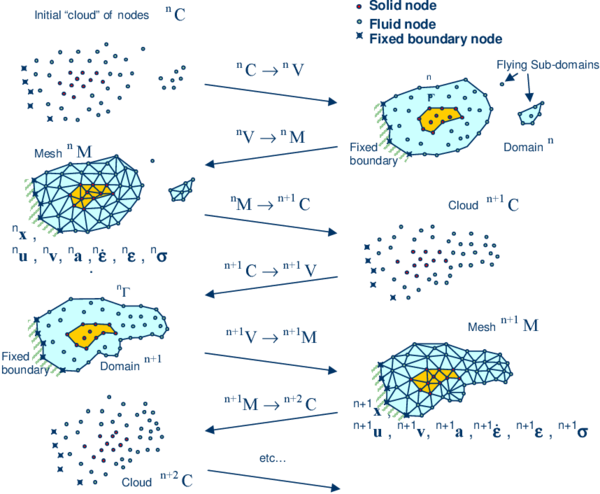Figure 1: Sequence of steps to update a “cloud” of nodes representing a domain containing a fluid and a solid part from time $n$ ($t=^{n}t$) to time $n+2$ ($t=^{n}t+2\Delta t$)
1. The starting point at each time step is the cloud of points ${\textstyle C}$ in the fluid and solid domains. For instance ${\textstyle ^{n}C}$ denotes the cloud at time ${\textstyle t={}^{n}t}$ (Figure 1).
2. Identify the boundaries defining the analysis domain ${\textstyle ^{n}V}$, as well as the subdomains in the fluid and the solid. This is an essential step as some boundaries (such as the free surface in fluids) may be severely distorted during the solution, including separation and re-entering of nodes. The Alpha Shape method  is used for the boundary definition. Clearly, the accuracy in the reconstruction of the boundaries depends on the number of points in the vicinity of each boundary and on the Alpha Shape parameter. In the problems solved in this work the Alpha Shape method has been implementation as described in [12,28].
3. Discretize the the analysis domain ${\textstyle ^{n}V}$ with a finite element mesh ${\textstyle {}^{n}M.}$We use an efficient mesh generation scheme based on an enhanced Delaunay tesselation [11,12].
4. Solve the Lagrangian equations of motion for the overall continuum using the standard FEM. Compute the state variables in at the next (updated) configuration for ${\textstyle ^{n}t+\Delta t}$: velocities, pressure and viscous stresses in the fluid and displacements, stresses and strains in the solid.
5. Move the mesh nodes to a new position ${\textstyle ^{n+1}C}$ where n+1 denotes the time ${\textstyle {}^{n}t+\Delta t}$, in terms of the time increment size.
6. Go back to step 1 and repeat the solution for the next time step to obtain ${\textstyle {}^{n+2}C}$.

Note that the key differences between the PFEM and the classical FEM are the remeshing technique and the identification of the domain boundary at each time step.

The CPU time required for meshing grows linearly with the number of nodes. As a general rule, meshing consumes for 3D problems around 15% of the total CPU time per time step, while the solution of the equations (with typically 3 iterations per time step) and the system assembly consume approximately 70% and 15% of the CPU time per time step, respectively. These figures refer to analyses in a single processor Pentium IV PC . Considerable speed can be gained using parallel computing techniques.

In this work we will apply the PFEM to problems involving a rigid domain containing fluid particles only. Application of the PFEM in fluid and solid mechanics and in fluid-structure interaction problems can be found in [4,5,6,8,9,10,11,12,13,14,15,16,17,18,19,28,29,35,36,39,40,41,42,43], as well in www.cimne.com/pfem.

## 8 EXAMPLES

### 8.1 Sloshing of water in prismatic tank

The problem has been solved first in 2D. Figure 2 shows the analysis data. The fluid oscillates due to the hydrostatic forces induced by its original position.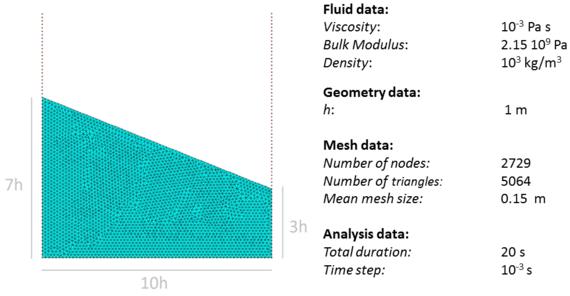Figure 2: 2D analysis of sloshing of water in rectangular tank. Initial geometry, analysis data and mesh of 5064 3-noded triangles discretizing the water in the tank

The problem has been run using different values of the parameter ${\textstyle \theta }$ in the tangent bulk stiffness matrix ${\textstyle {\bf {K}}_{v}^{e}}$ (Eq.(41)). The first set of results (Figures 3 and 4) were obtained with ${\textstyle \theta =1}$. The problem was then solved for ${\textstyle \theta =0.08}$, thereby, reducing in one order the magnitude the diagonal terms in ${\textstyle {\bf {K}}_{v}^{e}}$.

Figure 3 shows snapshots of the water geometry at different times. Pressure contours are superposed to the deformed geometry of the fluid in the figures.

Figure 4 shows the evolution of the percentage of water volume (i.e. mass) loss introduced by the numerical solution scheme. The accumulated volume loss (in percentage versus the initial volume) for the method proposed with ${\textstyle \theta =1}$ is approximately 1.33% over 20 seconds of simulation time (Figure 4a). The average volume variation in absolute value per time step is ${\textstyle 1.09\times 10^{-4}\%}$ (Figure 4b). The total water volume loss is the sum of the losses induced by the numerical scheme and the losses due to the updating of the free surface using the PFEM. No correction of mass was introduced at the end of each time step. Taking all this into account, the fluid volume loss over the analysis period is remarkably low.

The volume losses induced by the free surface updating can be reduced using a finer mesh in that region in conjunction with an enhanced alpha shape technique.

The total fluid volume loss can be reduced to almost zero by introducing a small correction in the free surface at the end of each time step .

The fluid volume losses obtained using a standard first order fractional step method  and the PFEM are shown in Figure 4a for comparison. Clearly the method proposed in this work leads to a reduction in the overall fluid volume loss, as well as in the volume loss per time step.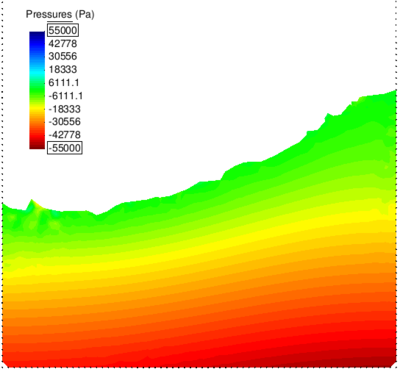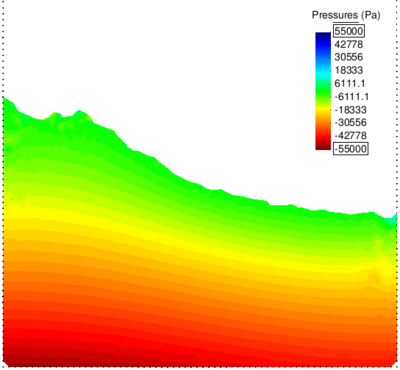(a) t=5.7s (b) t=7.4s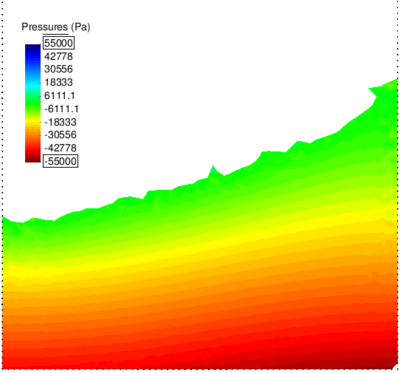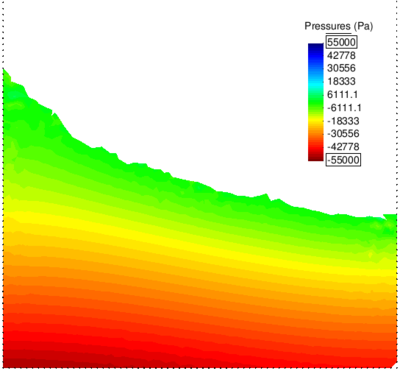(c) t=13.3s (d) t=18.6s Figure 3: 2D sloshing of water in rectangular tank. Snapshots of water geometry at two different times ($\theta =1$). Colours indicate pressure contours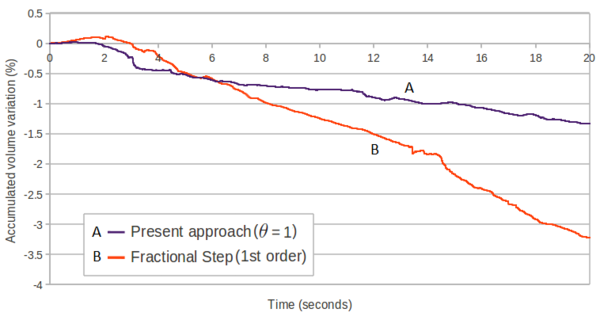(a) Accumulated volume loss over 20 seconds of analysis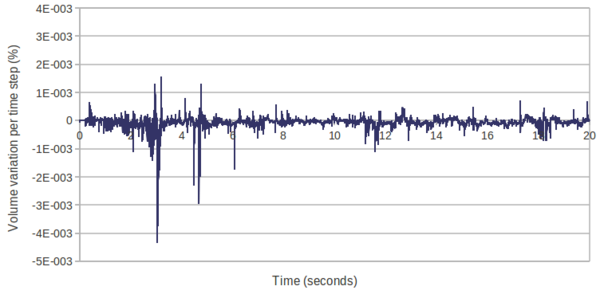(b) Volume variation (in %) per time step over 20 seconds of analysis (Current method with $\theta =1$) Figure 4: 2D sloshing of water in rectangular tank. (a) Time evolution of the percentage of water volume loss due to the numerical algorithm. (b) Average volume variation per time step. Current method. 1.09$\times 10^{-4}$% Fractional step: 2.07$\times 10^{-4}$%

Figure 5 shows a comparison between the fluid volume loss for ${\textstyle \theta =1}$ and ${\textstyle \theta =0.08}$ using the same time step in both cases (${\textstyle \Delta t=10^{-3}s}$). Results show that the reduction of the tangent bulk stiffness matrix terms leads to an improvement in the preservation of the initial volume of the fluid. It is noted that the convergence of the iterative solution for ${\textstyle \theta =0.08}$ was the same as for ${\textstyle \theta =1}$.

Figure 6 shows that a similar improvement in the volume preservation can be obtained using ${\textstyle \theta =1}$ and reducing the time step to ${\textstyle \Delta t=10^{-4}s}$. This, however, increases the cost of the computations.

These results indicate that accurate numerical results with reduced volume losses can be obtained by appropriately adjusting the parameter ${\textstyle \theta }$ in the tangent bulk modulus matrix while keeping the time step size to competitive values in terms of CPU cost. A study of the influence of ${\textstyle \theta }$ in the numerical solution for quasi-incompressible free surface fluids in terms of volume preservation and overall accuracy using the formulation here presented can be found in .

More results for this example can be found in .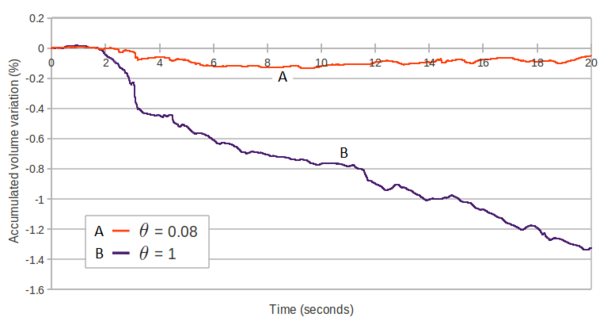Figure 5: 2D sloshing of water in rectangular tank. Time evolution of percentage of water volume loss obtained using the current method with $\theta =0.08$ (curve A) and $\theta =1$ (curve B) $\Delta t=10^{-3}s$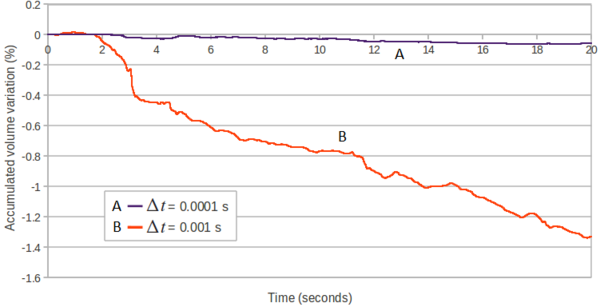Figure 6: 2D sloshing of water in rectangular tank. Time evolution of percentage of water volume loss obtained with the current method. Curve A: $\theta =1$ and $\Delta t=10^{-4}s$. Curve B: $\theta =1$ and $\Delta t=10^{-3}s$

Figures 7 and 8 show a similar set of results for the 3D analysis of the same sloshing problem using a relative coarse initial mesh of 106771 4-noded tetrahedra and ${\textstyle \theta =1}$. It is remarkable that the percentage of total fluid volume loss due to the numerical scheme after 10 seconds of analysis is approximately 1%.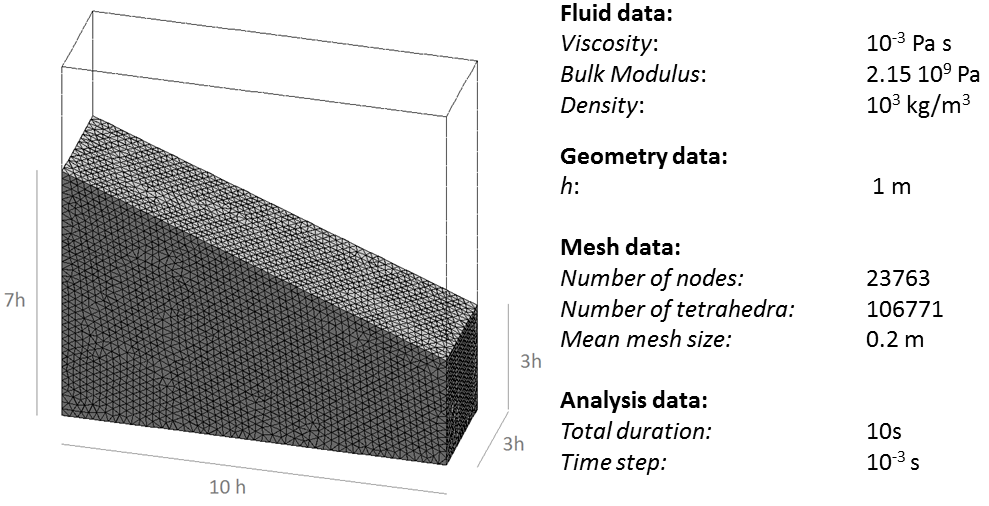]]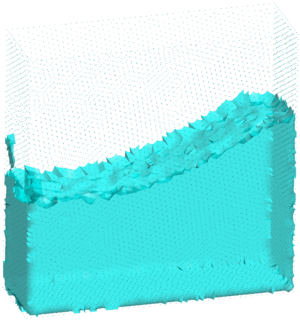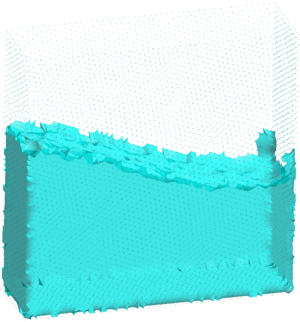(a) t=5.7s (b) t=7.4s Figure 7: 3D analysis of sloshing of water in prismatic tank ($\theta =1$). Analysis data and snapshots of water geometry at $t=5.7$s (a) and $t=7.4$s (b)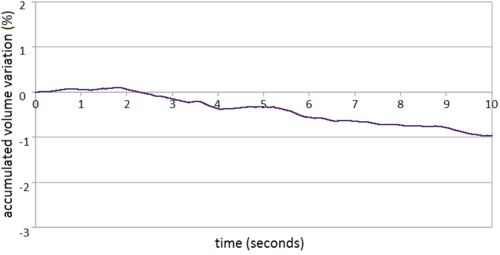(a)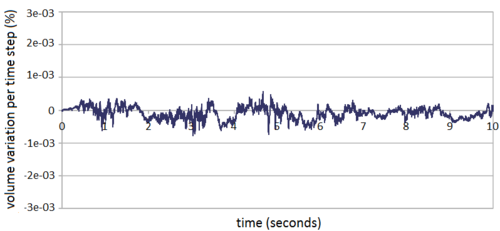(b) Figure 8: 3D analysis of sloshing of water in prismatic tank ($\theta =1$). (a) Time evolution of accumulated water volume loss (in percentage) due to the numerical algorithm. (b) Volume loss (in %) per time step over 2 seconds of analysis. Average volume loss per time step: 1.64$\times 10^{-4}$%

### 8.2 Falling of a water sphere in a cylindrical tank containing water

This example is the 3D analysis of the impact of a sphere made of water as it falls in a cylindrical tank containing water. Both the water in the sphere and in the tank mix in a single fluid after the impact. Figure 9 shows the material and analysis data and the initial discretization of the sphere and the water in the tank in 88892 4-noded tetrahedra. The problem was solved with the new stabilized method presented in the paper with ${\textstyle \theta =1}$. Figure 10 shows snapshots of the mixing process at different times. An average of four iterations for convergence of the velocity and the pressure were needed during all the steps of the analysis. The total water mass lost in the sphere and the tank due to the numerical algorithm was ${\textstyle \simeq }$ 2% after 3 seconds of analysis (Figure 11a).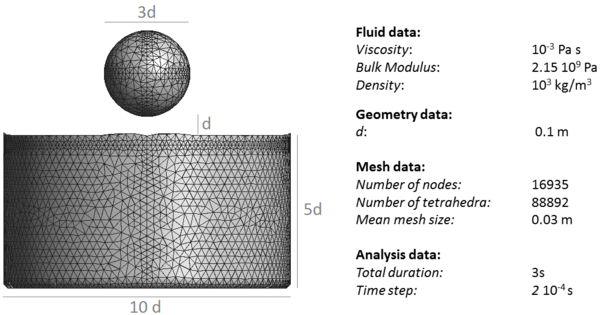Figure 9: Water sphere falling in a tank filled with water. Analysis data and initial discretization of the sphere and the water in the tank with 88892 4-noded tetrahedra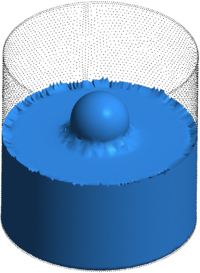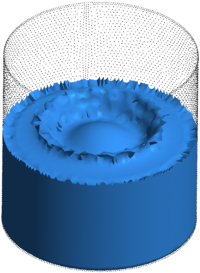(a) t=0.175s (b) t=0.275s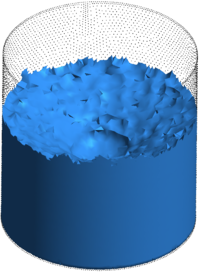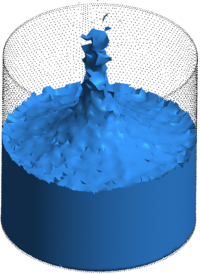(c) t=0.5s (d) t=0.9s Figure 10: Water sphere falling in tank containing water. Evolution of the impact and mixing of the two liquids at different times. Results for $\theta =1$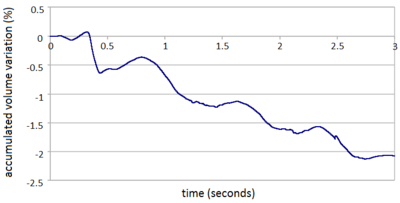(a)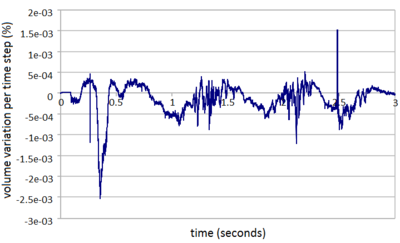(b) Figure 11: Water sphere falling in a tank containing water. (a) Accumulated volume over three seconds of analysis due to the numerical algorithm ($\theta =1$). (b) Volume loss (in %) per time step. Average volume variation per time step: 2.54$\times 10^{-4}$%

### 8.3 Sloshing of a fluid in a heated tank

A fluid at initial temperature ${\textstyle T=20\,^{\circ }C}$ oscillates due to the hydrostatic forces induced by its initial position in a rectangular tank heated to a uniform and constant temperature of ${\textstyle T=75\,^{\circ }C}$. The geometry and the problem data of the 2D simulation are shown in Figure 12. The fluid, with a very high thermal conductivity, changes its temperature only due to the contact with the hotter tank walls. The heat flux along the free surface has been considered null. The fluid domain has been initially discretized with 2828 3-noded triangles. The coupled thermal-fluid dynamics simulation has been run for 100 s using a time step increment of ${\textstyle \Delta t}$=${\textstyle 0.005s}$.

Figure 13 shows some snapshots of the numerical simulation. The temperature contours have been superposed on the fluid domain at the different time instants.

In Figure 14 the evolution of temperature with time at the points ${\textstyle A,B}$ and ${\textstyle C}$ of Figure 12 is plotted. The coordinates of these sample points are (${\textstyle m,0.1m}$), (${\textstyle m,0.4m}$) and (${\textstyle m,0.1m}$), respectively. Figures 13 and 14 show that the fluid does not heat uniformly because of the convection effect automatically captured by the Lagrangian technique here presented.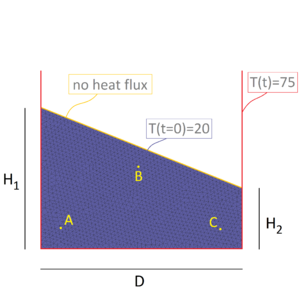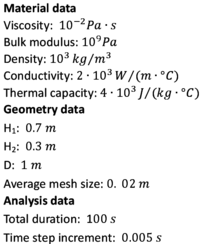Figure 12: 2D sloshing of a fluid in a heated tank. Initial geometry, problem data, thermal boundary and initial conditions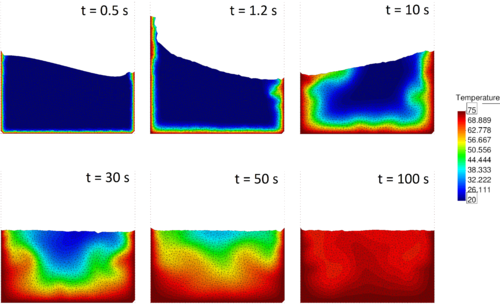Figure 13: 2D sloshing of a fluid in a heated tank. Snapshots of fluid geometry at six different times. Colours indicate temperature contours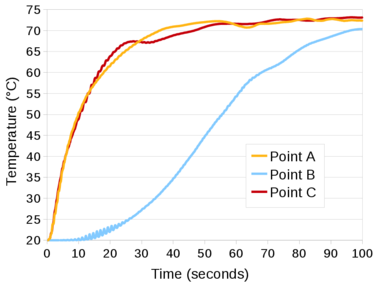Figure 14: 2D sloshing of a fluid in a heated tank. Evolution of temperature with time at the points $A,B$ and $C$ of Figure 12

### 8.4 Falling of a cylindrical object in a heated tank filled with fluid

An elastic object falls in a tank containing a fluid at rest. The tank walls are maintained at temperature ${\textstyle T}$=${\textstyle 75\,^{\circ }C}$ during the whole analysis, while the fluid and the solid object have an initial temperature of ${\textstyle T=20\,^{\circ }C}$. The geometry and the problem data of the 2D simulation as well the thermal initial and boundary conditions, are shown in Figure 15. Both the fluid and the solid have a high thermal conductivity. The heat flux along the fluid and solid surfaces in contact with the air has been considered null. The fluid and the solid domains have been discretized with 1986 and 108 3-noded triangular finite elements, respectively. The duration of the simulation is 10 s and the time step increment chosen is ${\textstyle \Delta t}$=${\textstyle 0.0005s}$.

Figure 16 collects some representative snapshots of the numerical simulation with the temperature results plotted over the fluid and the solid domains.

The graph of Figure 17 is the evolution of temperature at the central point of the solid object. As expected, its temperature tends to ${\textstyle T}$=${\textstyle 75\,^{\circ }C}$.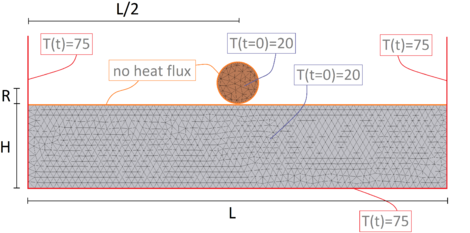Figure 15: Falling of a solid object in a heated tank filled with fluid. Initial geometry, problem data, thermal boundary and initial conditions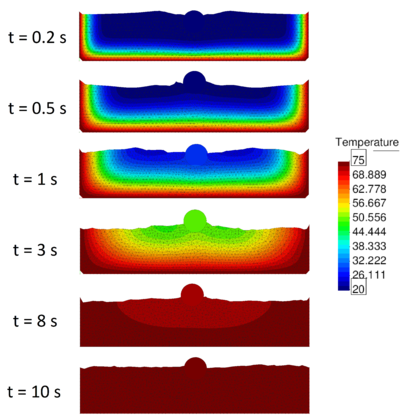Figure 16: Falling of a solid object in a heated tank filled with fluid. Snapshots at six different times. Colours indicate temperature contours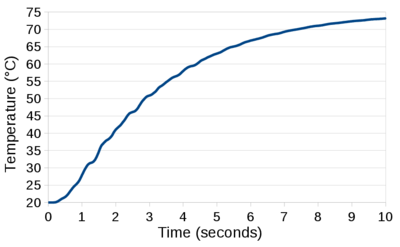Figure 17: Falling of a solid object in a heated tank filled with fluid. Time evolution of the temperature at the center of the solid.

## 9 CONCLUDING REMARKS

We have presented a new FIC-based stabilized Lagrangian finite element method for thermal-mechanical analysis of quasi and fully incompressible flows and FSI problems that has excellent mass preservation properties. The method has been successfully applied to the adiabatic and thermal-mechanical analysis of free-surface quasi-incompressible flows using the PFEM and an updated Lagrangian formulation. These problems are more demanding in terms of the mass preservation features of the numerical algorithm. The method proposed has yielded excellent results for 2D and 3D adiabatic and thermally-coupled free surface flow problems involving surface waves, water splashing, violent impact of flows with containment walls and FSI situations.

## ACKNOWLEDGEMENTS

This research was partially supported by the Advanced Grant project SAFECON of the European Research Council.

## Bibliography

 Aubry R, Idelsohn SR, Oñate E (2005) Particle finite element method in fluid-mechanics including thermal convection-diffusion. Computers and Structures, Vol. 83, (17-18), pp 1459–1475.

 Aubry R, Idelsohn SR, Oñate E (2006) Fractional step like schemes for free surface problems with thermal coupling using the Lagrangian PFEM. Computational Mechanics, Vol. 38 (4-5), pp. 294–309.

 Belytschko T, Liu WK, Moran B, Non linear finite element for continua and structures, 2d Edition, Wiley, 2013.

 Carbonell JM, Oñate E, Suárez B (2010) Modeling of ground excavation with the Particle Finite Element Method. Journal of Engineering Mechanics (ASCE) 136(4):455–463

 Carbonell JM, Oñate E, Suárez B (2013) Modelling of tunnelling processes and cutting tool wear with the Particle Finite Element Method (PFEM). Accepted in Comput. Mech. (2013) DOI:10.1007/s00466-013-0835-x

 Cremonesi M, Frangi A, Perego U (2011) A Lagrangian finite element approach for the simulation of water-waves induced by landslides. Computers & Structures 89:1086–1093

 Donea J, Huerta A (2003) Finite element method for flow problems. J. Wiley

 Edelsbrunner H, Mucke EP (1999) Three dimensional alpha shapes. ACM Trans. Graphics 13:43–72

 Felippa F, Oñate E (2007) Nodally exact Ritz discretizations of 1D diffusion-absorption and Helmholtz equations by variational FIC and modified equation methods. Comput. Mech. 39:91–111

 Franci A, Oñate E, Carbonell JM (2013) On the effect of the tangent bulk stiffness matrix in the analysis of free surface Lagrangian flows using PFEM. Research Report CIMNE PI402. Submitted to Int. J. Numer. Meth. Biomed. Engng.

 Idelsohn SR, Calvo N, Oñate E (2003c) Polyhedrization of an arbitrary point set. Comput. Method Appl. Mech. Engng. 192(22-24):2649–2668

 Idelsohn SR, Oñate E, Del Pin F (2004) The particle finite element method: a powerful tool to solve incompressible flows with free-surfaces and breaking waves. Int. J. Numer. Meth. Biomed. Engng., 61(7):964–989

 Idelsohn SR, Marti J, Limache A, Oñate E (2008) Unified Lagrangian formulation for elastic solids and incompressible fluids: Application to fluid-structure interaction problems via the PFEM. Comput Methods Appl Mech Engrg. 197:1762–1776

 Idelsohn SR, Mier-Torrecilla M, Oñate E (2009) Multi-fluid flows with the Particle Finite Element Method. Comput Methods Appl Mech Engrg. 198:2750–2767

 Idelsohn SR, Oñate E (2010) The challenge of mass conservation in the solution of free-surface flows with the fractional-step method: Problems and solutions. Int. J. Numer. Meth. Biomed. Engng. 26:1313–-1330

 Idelsohn SR, Nigro N, Limache A, Oñate E (2012) Large time-step explicit integration method for solving problem with dominant convection. Comput. Methods Appl. Mech. Engrg. 217-220:168–-185

 Larese A, Rossi R, Oñate E, Idelsohn SR (2008) Validation of the Particle Finite Element Method (PFEM) for simulation of free surface flows. Engineering Computations 25(4):385–425

 Limache A, Idelsohn, SR, Rossi R, Oñate E (2007) The violation of objectivity in Laplace formulation of the Navier-Stokes equations. Int. J. Numerical Methods in Fluids, 54:639–664.

 Oliver X, Cante JC, Weyler R, González C, Hernández J (2007) Particle finite element methods in solid mechanics problems. In: Oñate E, Owen R (Eds) Computational Plasticity. Springer, Berlin, pp 87–103

 Oñate E (1998) Derivation of stabilized equations for advective-diffusive transport and fluid flow problems. Comput. Meth. Appl. Mech. Engng. 151:233–267

 Oñate E, Manzan M (1999) A general procedure for deriving stabilized space-time finite element methods for advective-diffusive problems. International Journal for Numerical Methods in Fluids, Vol. 31, pp. 203–221.

 Oñate E (2000) A stabilized finite element method for incompressible viscous flows using a finite increment calculus formulation. Comput Methods Appl Mech Engrg. 182(1–2):355–370

 Oñate E, García J (2001) A finite element method for fluid-structure interaction with surface waves using a finite calculus formulation. Comput. Meth. Appl. Mech. Engrg. 191:635–660

 Oñate E (2003) Multiscale computational analysis in mechanics using finite calculus: an introduction. Comput. Meth. Appl. Mech. Engrg. 192(28-30):3043–3059

 Oñate E, Taylor RL, Zienkiewicz OC, Rojek J (2003) A residual correction method based on finite calculus. Engineering Computations 20:629–658

 Oñate E (2004) Possibilities of finite calculus in computational mechanics. Int. J. Num. Meth. Engng. 60(1):255–281

 Oñate E, Rojek J, Taylor R, Zienkiewicz O (2004a) Finite calculus formulation for incompressible solids using linear triangles and tetrahedra. Int. J. Numer. Meth. Engng. 59(11):1473–1500

 Oñate E, Idelsohn SR, Del Pin F, Aubry R (2004b) The particle finite element method. An overview. Int. J. Comput. Methods 1(2):267–307

 Oñate E, M.A. Celigueta, Idelsohn SR (2006a) Modeling bed erosion in free surface flows by the Particle Finite Element Method, Acta Geotechnia 1(4):237–252

 Oñate E, Valls A, García J (2006b) FIC/FEM formulation with matrix stabilizing terms for incompressible flows at low and high Reynold's numbers. Computational Mechanics 38 (4-5):440–455

 Oñate E, García J, SR Idelsohn, F. Del Pin (2006c) FIC formulations for finite element analysis of incompressible flows. Eulerian, ALE and Lagrangian approaches. Comput. Meth. Appl. Mech. Engng. 195(23-24):3001–3037

 Oñate E, Valls A, García J (2007) Computation of turbulent flows using a finite calculus-finite element formulation. Int. J. Numer. Meth. Engng. 54:609–637

 Oñate E, Idelsohn SR, Celigueta MA, Rossi R (2008) Advances in the particle finite element method for the analysis of fluid-multibody interaction and bed erosion in free surface flows. Comput. Meth. Appl. Mech. Engng. 197(19-20):1777–1800

 Oñate E (2009) Structural analysis with the finite element method. Linear statics. Volume 1. Basis and Solids. CIMNE-Springer

 Oñate E, Rossi R, Idelsohn SR, Butler K (2010) Melting and spread of polymers in fire with the particle finite element method. Int. J. Numerical Methods in Engineering, 81(8):1046–1072

 Oñate E, Celigueta MA, Idelsohn SR, Salazar F, Suárez B (2011) Possibilities of the particle finite element method for fluid-soil-structure interaction problems. Computational Mechanics, 48(3):307–318.

 Oñate E, Nadukandi P, Idelsohn SR, García J, Felippa C (2011) A family of residual-based stabilized finite element methods for Stokes flows. Int. J. Num. Methods in Fluids, 65 (1-3): 106–134

 Oñate E, Idelsohn SR, Felippa C (2011) Consistent pressure Laplacian stabilization for incompressible continua via higher-order finite calculus. Int. J. Numer. Meth. Engng, 87 (1-5): 171–195

 Oñate E, Nadukandi P, Idelsohn SR (2014) P1/P0+ elements for incompressible flows with discontinuous material properties. Comput. Meth. Appl. Mech. Engng. 271:185-209

 Oñate E, Carbonell JM (2013) Updated Lagrangian finite element formulation for quasi-incompressible fluids. Research Report PI393, CIMNE. Submitted to Comput. Mechanics

 Oñate E, Franci A, Carbonell JM (2013) Lagrangian formulation for finite element analysis of quasi-incompressible fluids with reduced mass losses. Int. J. Num. Meth. Fluids, DOI: 10.1002/fld.3870

 Ryzhakov P, Oñate E, Rossi R, Idelsohn SR (2012) Improving mass conservation in simulation of incompressible flows. Int. J. Numer. Meth. Engng. 90(12):1435–1451

 Tang B, Li JF, Wang TS (2009) Some improvements on free surface simulation by the particle finite element method. Int. J. Num. Methods in Fluids, 60 (9):1032–-1054

 Zienkiewicz OC, Taylor RL, Zhu JZ (2005) The finite element method. The basis, 6th Ed., Elsevier

 Zienkiewicz OC, Taylor RL (2005) The finite element method for solid and structural mechanics, 6th Ed., Elsevier

 Zienkiewicz OC, Taylor RL, Nithiarasu P (2005) The finite element method for fluid dynamics, 6th Ed., Elsevier

### Document informationPublished on 01/01/2014

DOI: 10.1007/978-3-319-06136-8

### Document Score0

Views 59
Recommendations 0